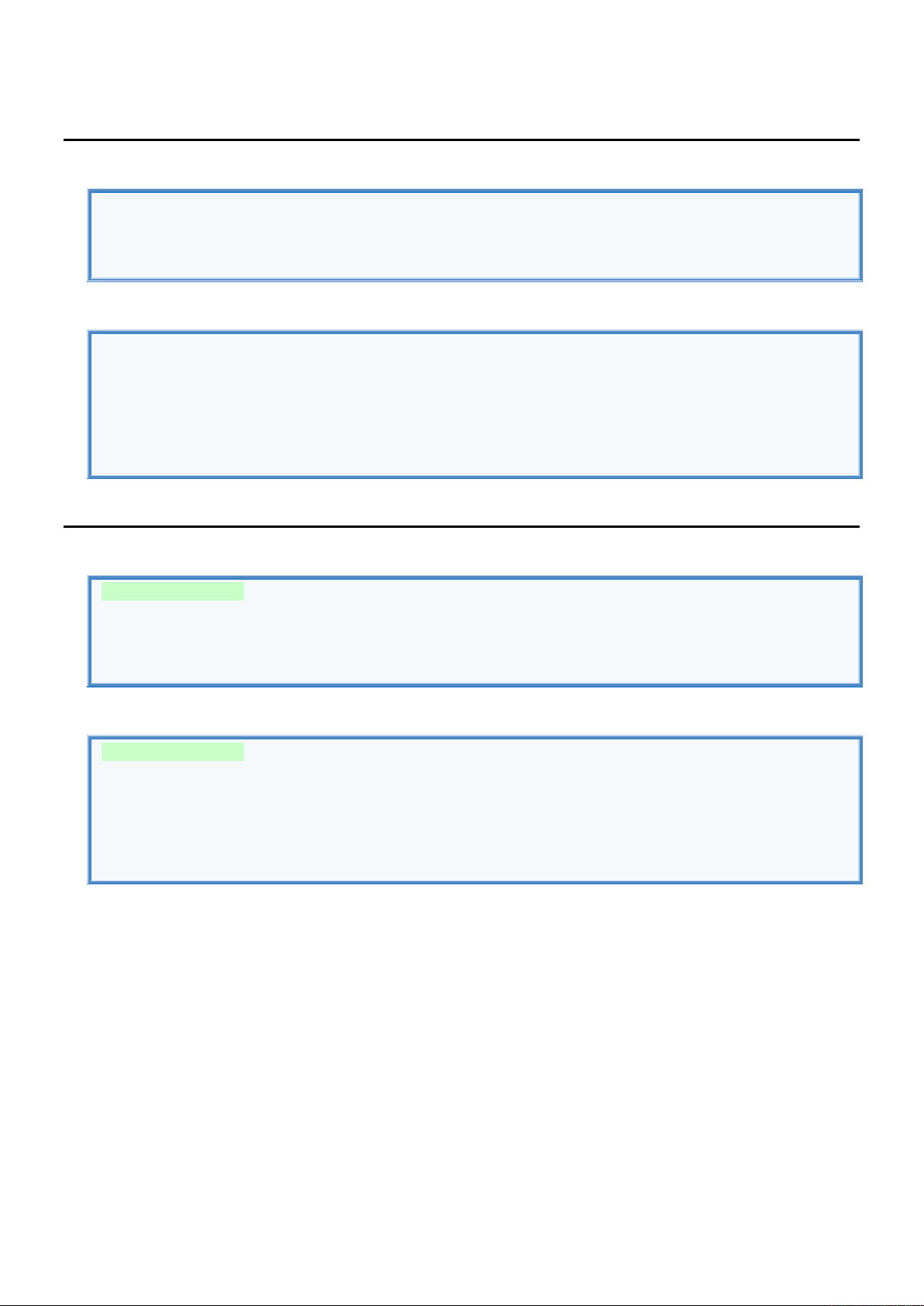3
3
.
.
3
3
B
B
a
a
s
s
i
i
c
c
S
S
y
y
n
n
t
t
a
a
x
x
C
C
o
o
m
m
m
m
e
e
n
n
t
t
s
s
&
&
P
P
r
r
i
i
n
n
t
t
//Single line comment.
/* Multi line
comment. */
Print
//DECLARE VARIABLES.
var name = "John"
var age = 20
//DISPLAY VARIABLES.
println("Hello" + name) //Hello John
print ("\${name} is \$age years old") //John is 20 years old
S
S
t
t
a
a
t
t
e
e
m
m
e
e
n
n
t
t
s
s
-
-
C
C
o
o
n
n
d
d
i
i
t
t
i
i
o
o
n
n
a
a
l
l
if
var result : String =
if (name == "John") "Name is John" //Can ommit {} for single line
else if (name == "Bill") "Name is Bill" //Don't use return to return value
else if (name == "Lucy") "Name is Lucy"
else { print("No match"); "No match found" }
when
var result : String =
when(name) {
"John" -> "Name is John" //Can ommit {} for single line
"Bill", "Lucy" -> { "Name is Bill or Lucy" } //It doesn't fall through to subsequent
case
else -> { print("No match"); " No match found" } //Don't use return to return value
}S
S
t
t
a
a
t
t
e
e
m
m
e
e
n
n
t
t
s
s
-
-
L
L
o
o
o
o
p
p
for
//ITERATE THROUGH SEQUENCE.
for (i in 1..5 step 2) { println(i) } // 1 3 5
for (i in 6 downTo 0 step 2) { println(i) } // 6 4 2 0
//ITERATE THROUGH ARRAY.
val people = arrayOf("John", "Bill", "Lucy") // DECLARE ARRAY
for (person in people ) { println(person) } // John Bill Lucy
for (index in people.indices ) { println(people[index]) } // John Bill Lucy
for ((index, person) in people.withIndex()) { println("\$index = \$person") } // 0 = John, 1 = Bill, 2 = Lucy
while
var i = 0
while(i < 5) {
i++
println(i)
}
do
var i = 0
do {
i++
println(i)
} while(i < 5)S
S
t
t
a
a
t
t
e
e
m
m
e
e
n
n
t
t
s
s
-
-
J
J
u
u
m
m
p
p
Break
//BREAK FROM NEAREST LOOP.
for(i in 1..5) {
if(i==3) { break } //Break from nearest Loop.
print(i) //1, 2
}
//BREAK FROM LABELED LOOP.
beginLoop@
for(i in 1..5) {
for(j in 10..15) {
if(j==13) { break@beginLoop } //Break from labeled Loop.
println(j) //10, 11, 12
}
}
Continue
//CONTINUE WITH NEAREST LOOP.
for(i in 1..5) {
if(i==3) { continue } //Continue with nearest Loop.
print(i) //1, 2, 4, 5
}
//CONTINUE WITH LABELED LOOP.
beginLoop@
for(i in 1..3) {
for(j in 10..15) {
if(j==13) { continue@beginLoop } //Continue with labeled Loop.
println(j) //(10, 11, 12) (10, 11, 12) (10, 11, 12) (10, 11, 12) (10, 11, 12)
}
}
Return
//DECLARE FUNCTION.
fun test() : String {
return("Hello from test.") //Return from the nearest enclosing function.
print("Never executed") //Skipped line.
}
//CALL FUNCTION.
var result = test() //Store function return value into variable result.
print(result)